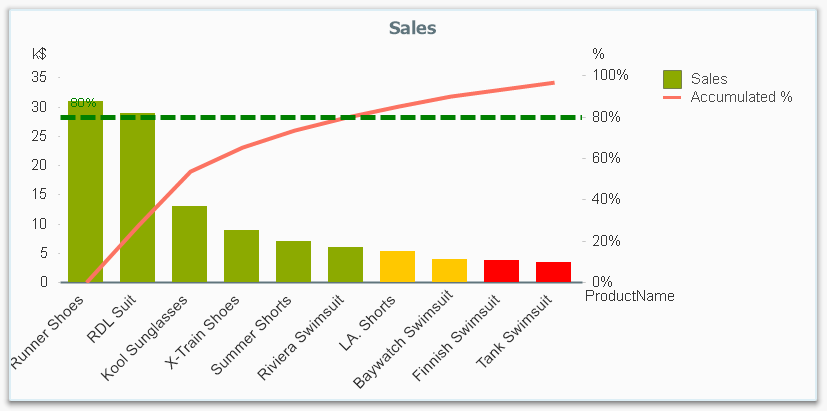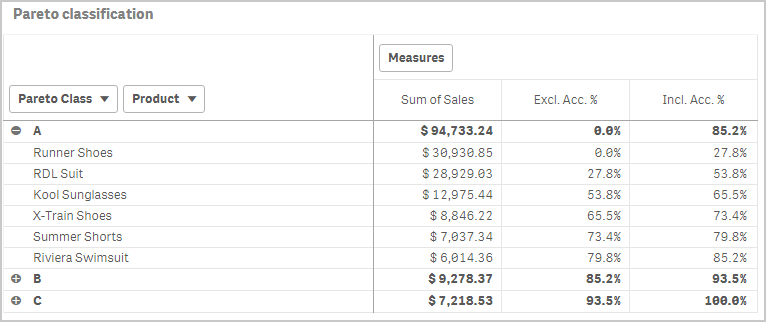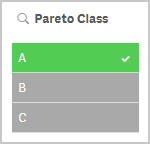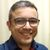# Qlik Design Blog

All about product and Qlik solutions: scripting, data modeling, visual design, extensions, best practices, etc.

Announcements
QlikWorld May 10-12, Online and Free! REGISTER NOWEmployee

## Recipe for a Pareto Analysis – Revisited

“Which products contribute to the first 80% of our turnover?”

This type of question is common in all types of business intelligence. I say “type of question” since it appears in many different forms: Sometimes it concerns products, but it can just as well concern any dimension, e.g. customer, supplier, sales person, etc. Further, here the question was about turnover, but it can just as well be e.g. number of support cases, or number of defect deliveries, etc.It is called Pareto analysis or ABC analysis and I have already written a blog post on this topic. However, in the previous post I only explained how to create a measure which showed the Pareto class. I never showed how to create a dimension based on a Pareto classification – simply because it wasn’t possible.

But now it is.

But first things first. The logic for a Pareto analysis is that you first sort the products according to their sales numbers, then accumulate the numbers, and finally calculate the accumulated measure as a percentage of the total. The products contributing to the first 80% are your best, your “A” products. The next 10% are your “B” products, and the last 10% are your “C” products. In the above graph, these classes are shown as colors on the bars.

The previous post shows how this can be done in a chart measure using the Above() function. However, if you use the same logic, but instead inside a sorted Aggr() function, you can achieve the same thing without relying on the chart sort order. The sorted Aggr() function is a fairly recent innovation, and you can read more about it here.

The sorting is needed to calculate the proper accumulated percentages, which will give you the Pareto classes. So if you want to classify your products, the new expression to use is

=Aggr(
If(Rangesum(Above(Sum({1} Sales)/Sum({1} total Sales),1,RowNo()))<0.8, 'A',
If(Rangesum(Above(Sum({1} Sales)/Sum({1} total Sales),1,RowNo()))<0.9, 'B',
'C')),
(Product,(=Sum({1} Sales),Desc))
)

The first parameter of the Aggr() – the nested If()-functions – is in principle the same as the measure in the previous post. Look there for an explanation.

The second parameter of the Aggr(), the inner dimension, contains the magic of the sorted Aggr():

(Product,(=Sum({1} Sales),Desc))

This structured parameter specifies that the field Product should be used as dimension, and its values should be sorted descending according to Sum({1} Sales). Note the equals sign. This is necessary if you want to sort by expression.

So the Products inside the Aggr() will be sorted descending, and for each Product the accumulated relative sales in percent will be calculated, which in turn is used to determine the Pareto classes.

The set analysis {1} is necessary if you want the classification to be independent of the made selection. Without it, the classification will change every time the selection changes. But perhaps a better alternative is to use {\$<Product=>}. Then a selection in Product (or in the Pareto class itself) will not affect the classification, but all other selections will.

The expression can be used either as dimension in a chart, or in a list box. Below I have used the Pareto class as first dimension in a pivot table.If you use this expression in a list box, you can directly select the Pareto class you want to look at.The other measures in the pivot table are the exclusive and inclusive accumulated relative sales, respectively. I.e. the lower and upper bounds of the product sales share:

Exclusive accumulated relative sales (lower bound):

=Min(Aggr(
Rangesum(Above(Sum({1} Sales)/Sum({1} total Sales),1,RowNo())),
(Product,(=Sum({1} Sales),Desc))
))

Inclusive accumulated relative sales (upper bound):

=Max(Aggr(
Rangesum(Above(Sum({1} Sales)/Sum({1} total Sales),0,RowNo())),
(Product,(=Sum({1} Sales),Desc))
))

Good luck in creating your Pareto dimension!

HIC

Further reading related to this topic:

The sortable Aggr function is finally here!

Recipe for a Pareto Analysis

Recipe for an ABC AnalysisMVP

Whoa! That's new isn't it? The help file still only mentions four Sort-types and Expression is not one of those for. So you can use an expression now to sort the aggr? That is awesome news.

So the number of products that generate 50% of the sales is:

Count(Distinct
Aggr(

If(Rangesum(Above(Sum({1} Sales)/Sum({1} total Sales),1,RowNo()))<0.5, Product),

(Product,(=Sum({1} Sales),Desc))

)
)

Very nice!

Hmm, but not exactly something a casual business user will cobble together. Perhaps we can make that easier with a variable and passing some parameters. A native function would be even better.

ParetoCount(Dimension, SortExpression, SortOrder, Fractile, InclusiveOrExclusive)

16,497 ViewsEmployee

To answer the question on your formula: Yes, it does exactly that.

And, no, a business user will probably not manage this. But it is still a step in the right direction, I think.HIC

16,497 ViewsMVP

Definitely a great step forward! And it isn't even X-mas yet. A late Sinterklaas surprise then16,497 ViewsLuminary Alumni

A hidden gem of the QIX Engine !

16,497 ViewsEmployee

Great!

A long-awaited feature

16,497 ViewsMVP

Nice one! But why it is a QlikView chart?

16,497 ViewsSpecialist III

Hi

What i tried to do was to have a dimension of customers (CSR) and a metric showing how many products made up 50% of the customers total sales

I started with this expression

Count({<DateType = {Invoice}, [Item Number] = >} DISTINCT

Aggr(

If(Rangesum(Above(Sum({<DateType = {Invoice}, [Item Number] = >}  [SalesUS\$])

/  Sum({<DateType = {Invoice},[Item Number] = >} TOTAL  [SalesUS\$]),1,RowNo()))<0.5,

[Item Number]),

([Item Number],(=Sum({<DateType = {Invoice}>}     [SalesUS\$]),Desc))

))

This only gave the correct figure if I filtered by just one customer (CSR)

So I tried different options. Nothing worked. Like

Count({<DateType = {Invoice}, [Item Number] = >} DISTINCT TOTAL <CSR,[Item Number]>

Aggr(

If(Rangesum(Above(Sum({<DateType = {Invoice}, [Item Number] = >} TOTAL <CSR,[Item Number]>   [SalesUS\$])

/  Sum({<DateType = {Invoice},[Item Number] = >} TOTAL <CSR>  [SalesUS\$]),1,RowNo()))<0.5,

[Item Number]),

CSR , ([Item Number],(=Sum({<DateType = {Invoice}>} TOTAL <CSR , [Item Number]>   [SalesUS\$]),Desc))

))

although filtering by just one customer always gave the correct total

I'm sure it can be done but my logic is a bit wrong

Thanks Robert

10,769 ViewsEmployee

I assume that you use this in a chart with Customer (CSR) as dimension. If so, the first expression will not work since CSR isn't used as dimension inside the Aggr().

In the second expression you have added CSR as inner dimension, but you have also added the Total qualifier in places where it shouldn't be used. You should have Total in the denominator inside the Aggr(), but not elsewhere.

HIC

10,769 ViewsSpecialist III

Thanks Henric. Including for this blog post

What I was doing was just trying every possible option I could think of. My first serious attempt was this one

Count({<DateType = {Invoice}, [Item Number] = >} DISTINCT

Aggr(

If(Rangesum (Above(Sum({<DateType = {Invoice}, [Item Number] = >}  [SalesUS\$])

/  Sum({<DateType = {Invoice},[Item Number] = >} TOTAL <CSR>   [SalesUS\$]),1,RowNo()))<0.9,

[Item Number]),

CSR , ([Item Number],(=Sum({<DateType = {Invoice}>}    [SalesUS\$]),Desc))

))

Its close but the figures are always either correct or a bit high. For example the first 4 lines should be 8,61,34,15 not 9,82,41,15. I will continue working on it tomorrow. A number of clients have asked for this sort of report and I had to do it in a two step process before (download then upload). But it will be great if I can work this out.

First column = customer10,769 ViewsEmployee

I just realised that this probably isn't possible after all.

You want to sort the Aggr() the following way:

1. By CSR according to alphabet
2. By Item according to Sum(Sales) desc

However, bullet two will always be sorted globally, i.e. there can only be one sort order for the dimension "Item". But you want different sort orders for different customers (CSR), and this is not possible.

HIC

10,769 Views